### Home > A2C > Chapter Ch11 > Lesson 11.3.1 > Problem11-132

11-132.
1. For the function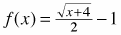, complete parts (a) through (d) below. Homework Help ✎

1. Sketch the graph and the inverse.

2. Find the equation of the inverse function.

3. Determine the domain and range of the inverse.

4. Compute f −1(f (5)).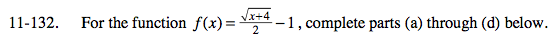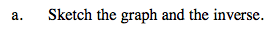First, graph the function. Then, reflect the graph across
y = x.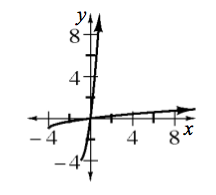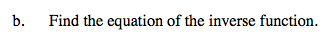Replace f(x) with y, then switch the variables.

$x=\frac{\sqrt{y+4}}{2}-1$

Now solve for y.

Do not forget to replace y with f − 1(x).

f −1(x) = (2(x + 1))2 − 4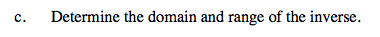See graph in part (a).

x ≥ −1, y ≥ −4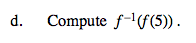Substitute 5 for x in the function f(x). Now substitute the answer you found for f(x) for x in the inverse function
f −1(x).

f −1(f(5)) = 5